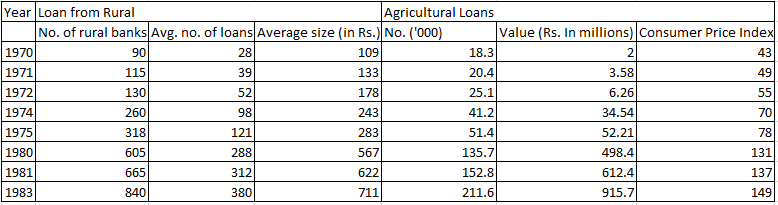### CAT 1997 Question Paper Question 25

Instructions

The table given below gives the annual details of loans from rural banks and agricultural loans over the years 1970 to 1983. Using this data answer the questions that follow.Question 25

# Which of the following pairs of years showed the maximum increase in the number of rural bank loans?

Solution

Number of loans in 1970 = 90 * 28 = 2520

Number of loans in 1971 = 115*39 = 4485

Number of loans in 1972 = 130 * 52 = 6760

Number of loans in 1974 = 260 * 98 = 25480

Number of loans in 1975 = 318 * 121 = 38478

Number of loans in 1980 = 605 * 288 = 174240

Number of loans in 1981 = 665 * 312 = 207480

So, the maximum increase in number of loans is in 1980 - 81